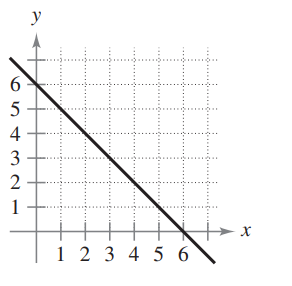×
Get Full Access to Calculus: Early Transcendental Functions - 6 Edition - Chapter 1.2 - Problem 3
Get Full Access to Calculus: Early Transcendental Functions - 6 Edition - Chapter 1.2 - Problem 3

×

# ?In Exercises 1–4, estimate the slope of the line from its graph. To print an enlarged copy of the graph, go to MathGraphs.com.ISBN: 9781285774770 141

## Solution for problem 3 Chapter 1.2

Calculus: Early Transcendental Functions | 6th Edition

• Textbook Solutions
• 2901 Step-by-step solutions solved by professors and subject experts
• Get 24/7 help from StudySoup virtual teaching assistantsCalculus: Early Transcendental Functions | 6th Edition

4 5 1 267 Reviews
24
2
Problem 3

In Exercises 1–4, estimate the slope of the line from its graph. To print an enlarged copy of the graph, go to MathGraphs.com.Step-by-Step Solution:

Step 1 of 5) Solution Using Figure 11.13(a), represent the velocity of the airplane (alone) as

Step 2 of 2

## Discover and learn what students are asking

Statistics: Informed Decisions Using Data : Introduction to the Practice of Statistics
?Explain the difference between a population and a sample.

Unlock Textbook Solution